Click in-app to access the full platform documentation for your version of DataRobot.

# Confusion Matrix (for multiclass models)¶

For multiclass models, DataRobot provides a multiclass confusion matrix to help evaluate model performance. The confusion matrix compares actual data values with predicted data values, making it easy to see if any mislabeling has occurred and with which values.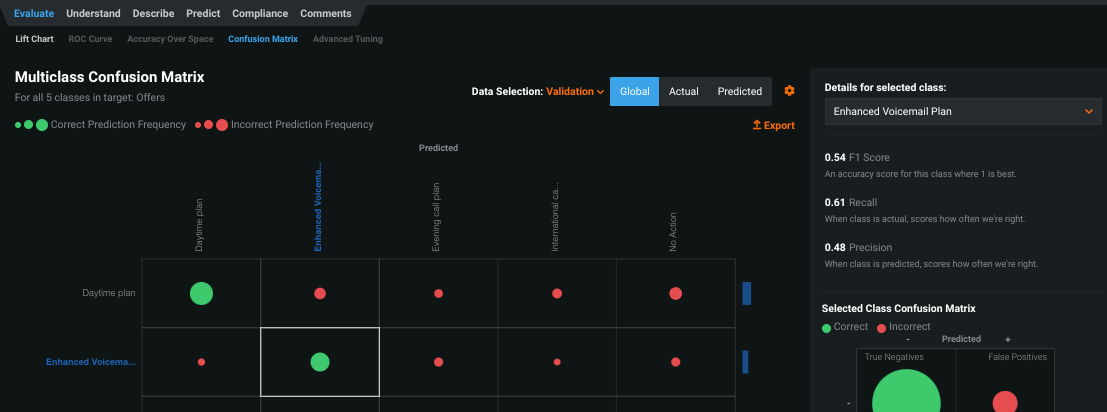## Background¶

In general, there are two types of prediction problems—regression and classification. Regression problems predict continuous values (1.7, 6, 9.8…). Classification problems, by contrast, classify values into discrete, final outputs or classes (buy, sell, hold...).

Classification can be broken down into binary and multiclass problems. In a binary classification problem, there are only two possible classes. Some examples include predicting whether or not a customer will pay their bill on time (yes or no) or if a patient will be readmitted to the hospital (true or false).

Multiclass classification problems, on the other hand, answer questions that have more than two possible outcomes (classes). For example, which of five competitors will a customer turn to (instead of simply whether or not they are likely to make a purchase). Or, to which department should a call be routed (instead of simply whether or not someone is likely to make a call)? With additional class options for multiclass classification problems, you can ask more “which one” questions, which result in more nuanced models and solutions.

## Work with multiclass models¶

DataRobot supports both binary and multiclass classification, each using the same general model building workflow. Depending on the number of values for a given target feature, DataRobot automatically determines the project type and whether a project is standard or extended multiclass. The following table describes how DataRobot assigns a default problem type for numeric and non-numeric target data types:

Target data type Number of values Default problem type Use multiclass?
Numeric 3-10 Regression Yes, optional
Numeric > 10 Regression Yes, optional (extended multiclass)
Non-numeric 2 Binary No
Non-numeric 3-100 Multiclass Yes, automatic

To begin building multiclass models, first import a dataset and when complete, specify a target.

The target field displays the selected target feature and one of DataRobot’s default model training methods: regression (numeric) or classification (non-numeric).

### Change regression projects to multiclass¶

Once you enter a target feature, DataRobot classifies the project type and indicates the default with a tag next to the target feature: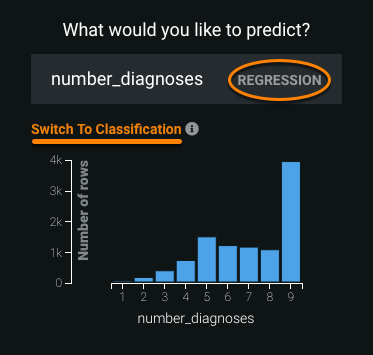If the project is classified as regression, and eligible for multiclass conversion, DataRobot provides a Switch To Classification link below the target entry box. Clicking the link changes the project to a classification project (values are interpreted as classes instead of continuous values). If the number of unique values falls outside the allowable range, the Switch To Classification link is not available.

Click Switch To Regression to switch the project type from classification back to the default regression setting.

With the training method set, verify or change the metric, choose a modeling mode, and click Start.

## Confusion Matrix tab¶

For each classification project type, DataRobot builds a confusion matrix to help evaluate model performance. The name "confusion matrix" refers to how a model can confuse two or more classes by consistently mislabeling (confusing) one class as another. The confusion matrix compares actual data values with predicted data values, making it easy to see if any mislabeling has occurred and with which values.

A confusion matrix specific to the problem type is available for both binary (in the ROC Curve) and multiclass problems. To access the multiclass confusion matrix, first build your models and then select the Confusion Matrix tab from the Evaluate division.

The tab displays two confusion matrix tables for each multiclass model: the Multiclass Confusion Matrix and the Selected Class Confusion Matrix. Both matrices compare predicted and actual values for each class, which are based on the results of the training data used to build the project, and through the graphic elements illustrate mislabeling of classes. The Multiclass Confusion Matrix provides an overview of every class found for the selected target, while the Selected Class Confusion Matrix analyzes a specific class. From these comparisons, you can determine how well DataRobot models are performing.

The following describes the components available in the Confusion Matrix tab.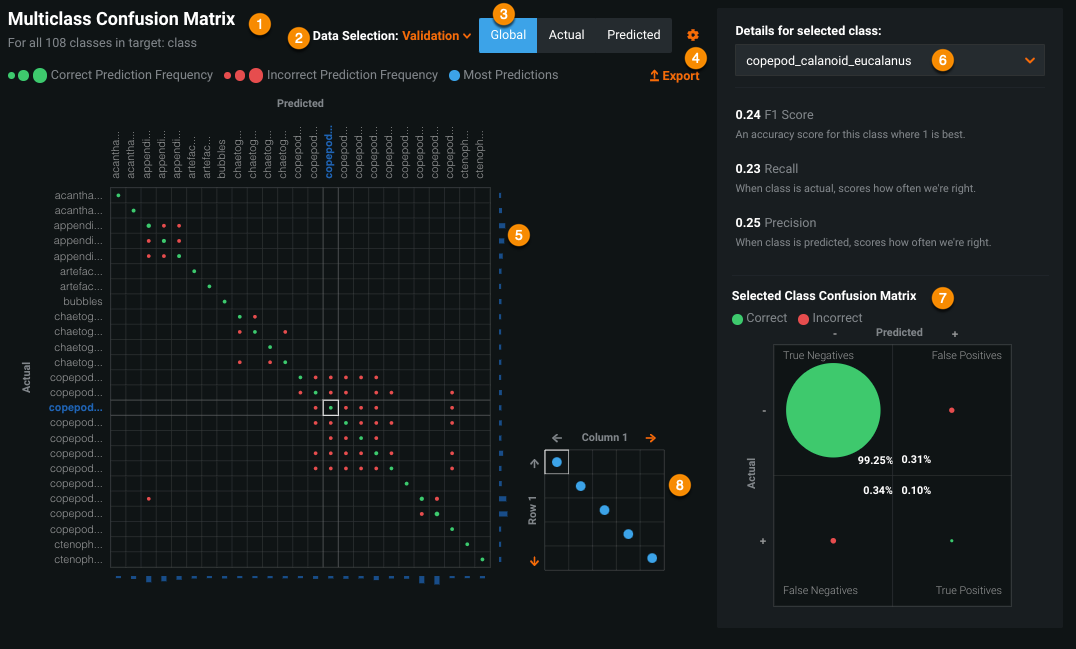Option DescriptionMatrix Overview of every found class.Source Data partition used.Modes Modes that impact display.Display options Menu for display options.Matrix detail Numeric frequency details.Class selector Individual class selector.Selected Class Confusion Matrix Class-specific matrix.Extended-class Confusion Matrix thumbnail Thumbnail for extended classes.

### Multiclass Confusion Matrix¶

This matrix provides an overview of every class (value) that DataRobot recognized for the selected target in the dataset. It reports class prediction results using different colored and sized circles. Color indicates prediction accuracy—green circles represent correct predictions while red circles represent incorrect predictions. The size of a circle is a visual indicator of the occurrence (based on row count) of correct and incorrect predictions (for example, the number of rows in which “product problem” was predicted but the actual value was “bad support”).

Click on any of the correct predictions (green circles) in the Multiclass Confusion Matrix to view and analyze additional details for that class in the display to the right of the matrix.

### Source¶

The data used to build the Multiclass Confusion Matrix is sourced from the validation, cross-validation, or holdout (if unlocked) partitions—subsets of your historical (training) data. You can change the source of the data that DataRobot uses in this confusion matrix by selecting from the Source dropdown.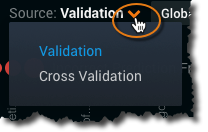### Modes¶

There are three mode options—Global, Actual, and Predicted—that provide detailed information about each class within the target column. Changing the mode updates the full matrix, the selected class matrix, and the details for the selected class.The following table describes each of the Multiclass Confusion Matrix modes.

Mode Description Hover over a cell on the matrix grid to display...
Global Provides F1 Score, Recall and Precision metrics for each selected class.
• total row count
• total row count compared to total row count in the selected partition (%)
Actual Provides details of the Recall score as well as a partial list of classes that the model confused with the selected class. Click Full List to see Recall score for all confused classes.*
• total row count
• total row count compared to the total row count of actual class values in the selected partition (%)
Predicted Provides details of the Precision score (how often the model accurately predicted the selected class). Click Full List to see Precision score for all confused classes.*
• total row count
• total row count compared to the total row count of predicted class values in the selected partition (%)

Clicking Full List opens the Feature Misclassification popup, which lists scores for all classes and allows you to switch between the Actual and Predicted modes.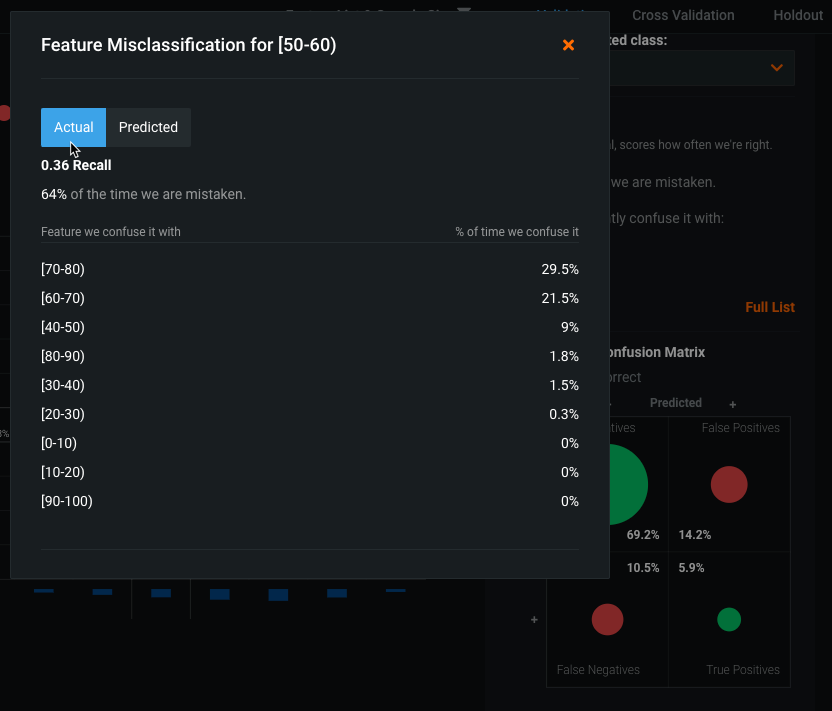### Display options¶

The gear icon provides a menu of options for sorting and orienting the Multiclass Confusion matrix into different formats.Display options include:

• Orientation of Actuals: sets the axis (rows or columns) for the Actual values display.
• Sort by: sets the sort order, either alphabetically, by actual or predicted frequency, or by F1 Score.
• Order: orders the matrix display in either ascending or descending order.

For example, to view the lowest Predicted Frequency values, select the Predicted Frequency and Ascending order options to display those values at the top of the matrix.

### Matrix detail¶

The blue bars that border the right and bottom sides of the Multiclass Confusion Matrix display numeric frequency details for each class and help determine DataRobot’s accuracy. For any class, click a bar across opposite the Actual axis to see actual frequency, or opposite the Predicted axis to see predicted frequency.

The example below reports the actual frequency for the class `[50-60)` of the feature `age`. In this case, based on the training data, there were 264 instances (at this sample size) in which the `[50-60)` class was the value of the target `age`. Those 264 rows make up 16.5% of the total dataset: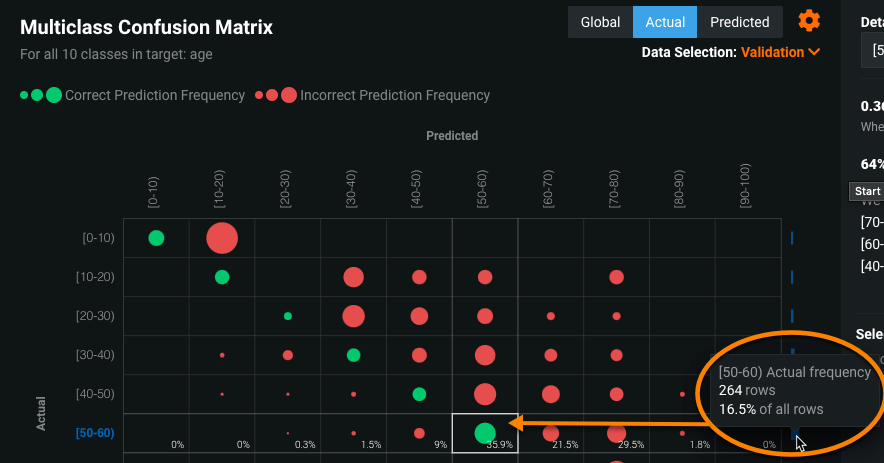Tip

You can view frequency details for any class, regardless of which class is currently selected, by hovering over any of the blue bars.

### Class selector¶

The dropdown selects an individual class and provides details based on the active mode.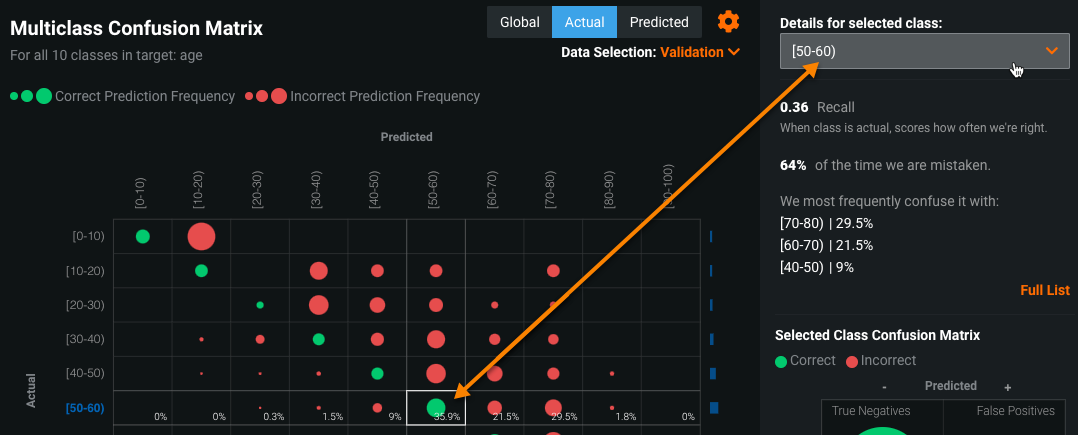### Selected Class Confusion Matrix¶

The smaller matrix provides accuracy details for a a single class. Changing the mode or the selected class, whether through the dropdown or by clicking a green circle in the full matrix, dynamically updates the Selected Class Confusion Matrix. The class displayed on the Selected Class Confusion Matrix is simultaneously highlighted on the full matrix and the frequency percentages are displayed in the labeled quadrants. Hover over a circle in the matrix to view its contribution to the total number of rows in that sample (for the selected partition). The sum of rows in each quadrant equals the total dataset. For example, there are 1600 instances where `Bad Support` was the value of the target ChurnReasons. Hover over each quadrant to view a count of each outcome (the accuracy) of the DataRobot prediction.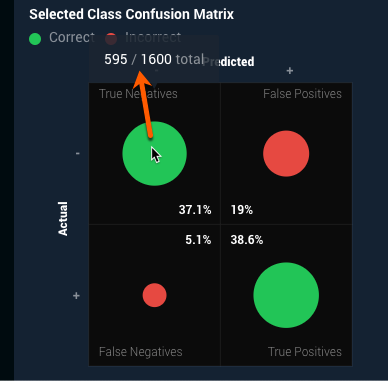The Selected Class Confusion Matrix is divided into four quadrants, summarized in the following table:

True Positive For all rows in the dataset that were actually ClassA, how many (what percent) did DataRobot correctly predict as ClassA? This quadrant is equal to the value reflected in the full matrix.
True Negative For all rows in the dataset that were not ClassA, how many (what percent) did DataRobot correctly predict as not ClassA? This quadrant is equal to the value reflected in the full matrix.
False Positive For all rows in the dataset that DataRobot predicted as ClassA, how many (what percent) were not ClassA? This is the sum of all incorrect predictions for the class in the full matrix.
False Negative For all rows in the dataset that were ClassA, how many (what percent) did DataRobot incorrectly predict as something other than ClassA? This quadrant shows the sum of all rows that should have been the selected class in the full matrix but were not.

### Extended-class Confusion Matrix thumbnail¶

For extended-class (between 11 and 100) multiclass projects, DataRobot provides a thumbnail pagination tool to allow you a more detailed inspection of your results. The thumbnail is a smaller representation of the full multiclass matrix. The blue dots in the thumbnail indicate locations that contain the most predictions (whether classified correctly or incorrectly) and therefore might be the most interesting to investigate.

Clicking on an area in the thumbnail updates the larger matrix to display the 10x10 area surrounding your selection. The final frame (lower right corner) displays only the remaining columns beyond the last `10` boundary (for example, a dataset with 83 classes will show only three entries). The full matrix functions in the same way as the non-extended multiclass matrix described above. Statistics on each cell shown in the larger 10x10 matrix are calculated across the full confusion matrix represented by the thumbnail.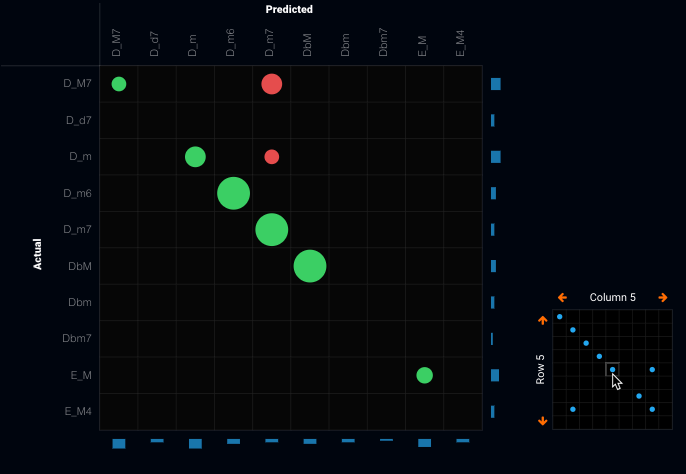You can navigate the thumbnail either using the arrows along the outside or by clicking in a specific box; row and column numbers help identify the current matrix position: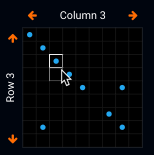A thumbnail displaying blue dots roughly on the diagonal from upper left to lower right potentially indicates a good model—there are many correct predictions. However, it is also possible that, because categories are not ordered, the dots indicate misses that are gathered by chance and so it is important to fully investigate each square to check performance.

Updated September 14, 2021